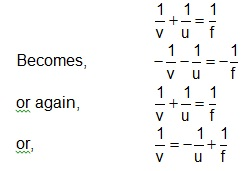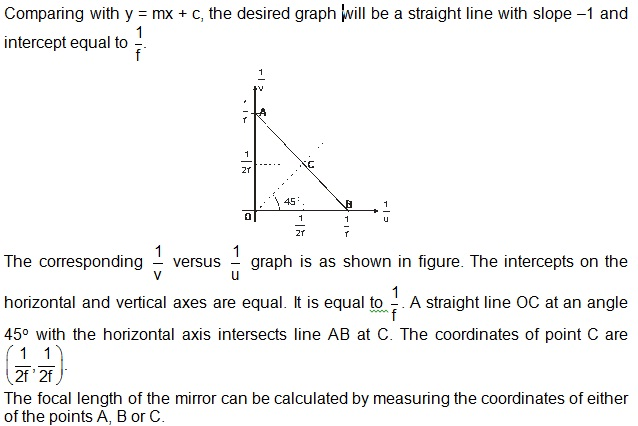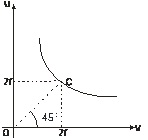# Determination of Focal Length of a Concave Mirror by u–v Method

The relation between object distance u and the image v from the pole of the mirror is given by,
v-1  + u-1 = f-1

Where, f is the focal length of the mirror. The focal length of the concave mirror is obtained either from  v-1 versus u-1 graph or from u–v graph.

When the image is real (of course only upon then it can be obtained on screen), the object lies between focus (F) and infinity. In such a situation u, v and f all are negative. Hence the mirror formulaA line drawn at angle 45o from the origin intersects the hyperbola at point C. The coordinates of point C are (2f, 2f).
The focal length of mirror can be calculated by measuring the coordinates of point C.To read more, Buy study materials of Ray Optics and Optical Instruments comprising study notes, revision notes, video lectures, previous year solved questions etc. Also browse for more study materials on Physics here.### Course Features

• Video Lectures
• Revision Notes
• Previous Year Papers
• Mind Map
• Study Planner
• NCERT Solutions
• Discussion Forum
• Test paper with Video Solution